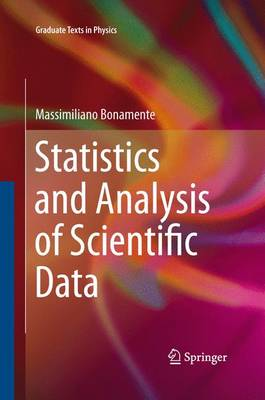•Statistics and Analysis of Scientific Data - Graduate Texts in Physics (Paperback)

(author)
£59.99
Paperback 301 Pages / Published: 17/09/2015
• We can order this

Usually dispatched within 3 weeks

Statistics and Analysis of Scientific Data covers the foundations of probability theory and statistics, and a number of numerical and analytical methods that are essential for the present-day analyst of scientific data. Topics covered include probability theory, distribution functions of statistics, fits to two-dimensional datasheets and parameter estimation, Monte Carlo methods and Markov chains. Equal attention is paid to the theory and its practical application, and results from classic experiments in various fields are used to illustrate the importance of statistics in the analysis of scientific data.

The main pedagogical method is a theory-then-application approach, where emphasis is placed first on a sound understanding of the underlying theory of a topic, which becomes the basis for an efficient and proactive use of the material for practical applications. The level is appropriate for undergraduates and beginning graduate students, and as a reference for the experienced researcher. Basic calculus is used in some of the derivations, and no previous background in probability and statistics is required. The book includes many numerical tables of data, as well as exercises and examples to aid the students' understanding of the topic.

Publisher: Springer-Verlag New York Inc.
ISBN: 9781489994806
Number of pages: 301
Weight: 4861 g
Dimensions: 235 x 155 x 17 mm
Edition: Softcover reprint of the original 1st ed. 201